# Why does the price input automatically round?

#### When providing liquidity using Uniswap v3, your input price will always round to the nearest tick.

This is because Uniswap v3 divides prices up into intervals known as ticks.

The size of tick spacing is relative to the fee tier. The tick spacing is double the size of the fee tier. For example, a tick for 0.30% fee tier pool will be 0.60% in size.

This spacing does not apply to the 0.01% fee tier. The 0.01% fee tier has a size of 0.01%.

There is a tick for every price 1.0001n, where n is an integer. This is the number generally seen quoted on exchanges and data sources. This would be the price of 1 token relative to a single token of the other.

Here are the pricing formulas: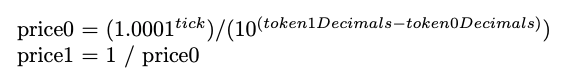Here is an example of how the price is rounded to the nearest tick:

A liquidity provider (LP) wishes to deposit into the ETH/USDC pool with:

• 0.30% fee tier
• price range of 1,000 to 5,000 USDC per ETH

The nearest low price tick for the \$1000 would be 207240.*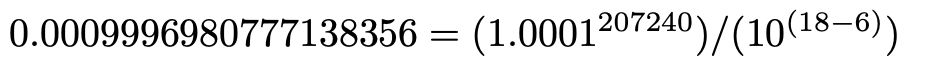We then use the price1 formula from above to get USDC price of 1000.302013.

The nearest high price tick for the \$5000 would be 191160.*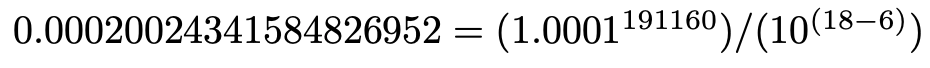We then use the price1 formula from above to get the USDC price of 4993.922001.

With these price inputs of 1,000 and 5,000, the liquidity positions price inputs will round to 1000.3 and 4,993.92 USDC per ETH.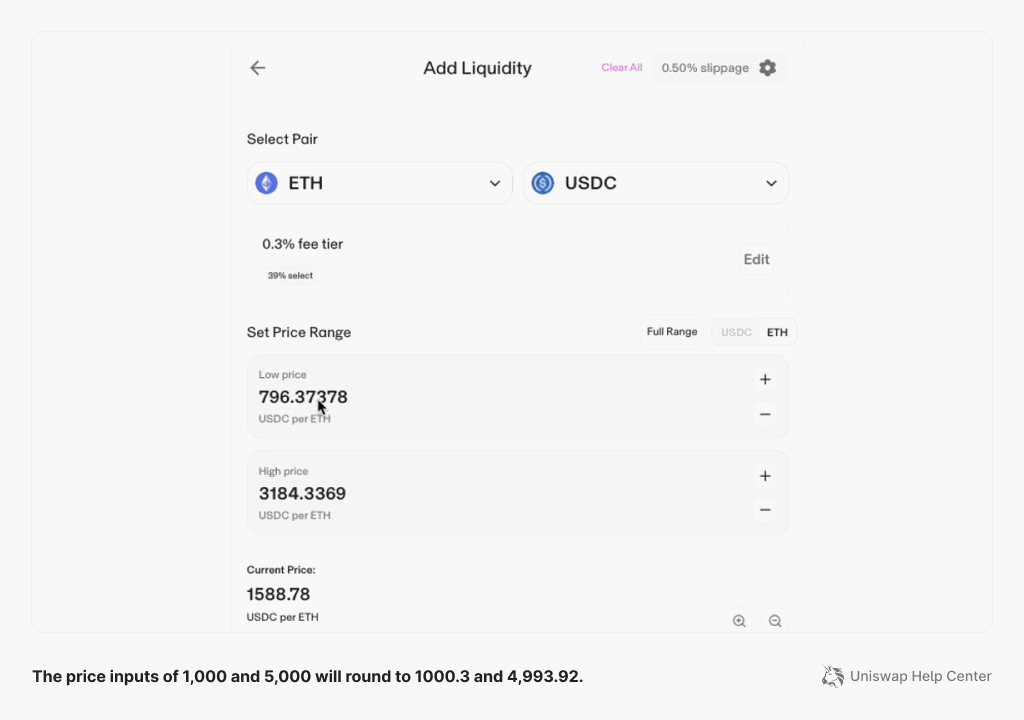*This number was calculated using this formula.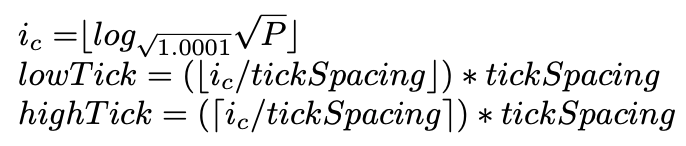Updated